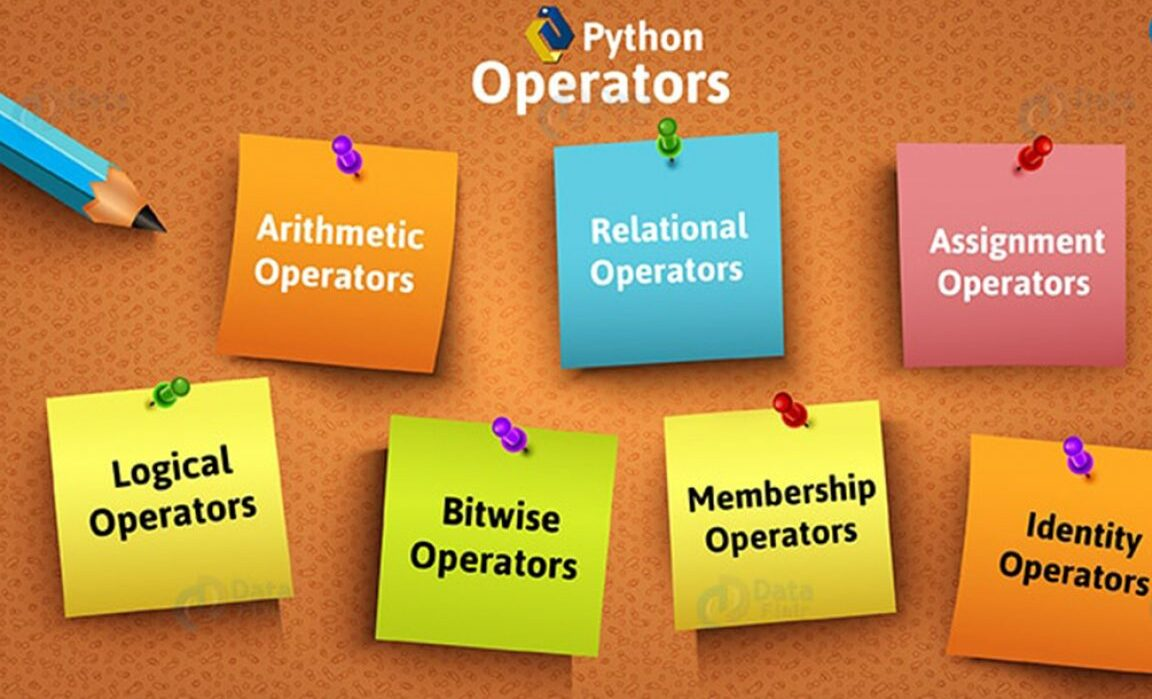# BASIC OPERATORS IN PYTHON – 1## OVERVIEW

In this blog, you will learn all about the different types of operators in python. Which are:

• Arithmetic Operators
• Comparison(Relational) Operators
• Membership Operators
• Logical Operators
• Bitwise Operators
• Identity Operators
• Assignment operators

Now let’s understand about them one by one.

## Example

Assume variable a holds 10 and variable b holds 20, then-

When you execute the above program arithmetic operators in python, it returns the following result-

## Example

Assume variable a holds 10 & variable b holds 20, then-

When you execute the above program comparison operators in python, it returns the following result-

## Example

Assume variable a holds 10 and variable b holds 20, then-

When you execute the above program assignment operators in python, it returns the following result-

## BITWISE OPERATORS IN PYTHON

Bitwise operators works bit wise and performs operation bit-by-bit. Assume a = 60 and b = 13. Now in binary format they will be as follows-

a = 0011 1100
b = 0000 1101
———————-
a&b = 0000 1100
a|b = 0011 1101
a^b = 0011 0001
~a = 1100 0011

Python’s built-in function bin() can be used to obtain binary representation of an integer
number.

## Example

When you execute the above program bitwise operators in python, it returns the following result-

## LOGICAL OPERATORS IN PYTHON

The following logical operators in python are supported by Python language. Assume variable a has value True and variable b has value False then-

## Example

When you execute the above program for membership operators in python, it returns the following result-

## Example

When you execute the above program identity operators in python, it returns the following result-

Check out this video for more clarification:

Time to wrap up now. See you in our next blog, thanks for reading our blog and do leave a comment below to help us improve the content to serve you all of our experience with you. Stay tuned with us for more python programming contents.

Also check out our other playlist Rasa Chatbot, Internet of things, Docker, etc.
Become a member of our social family on youtube here.